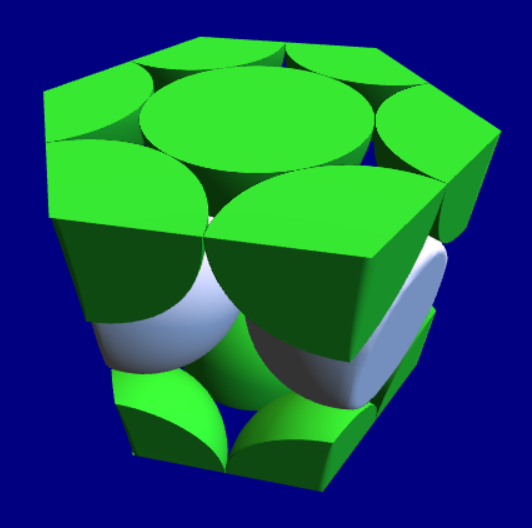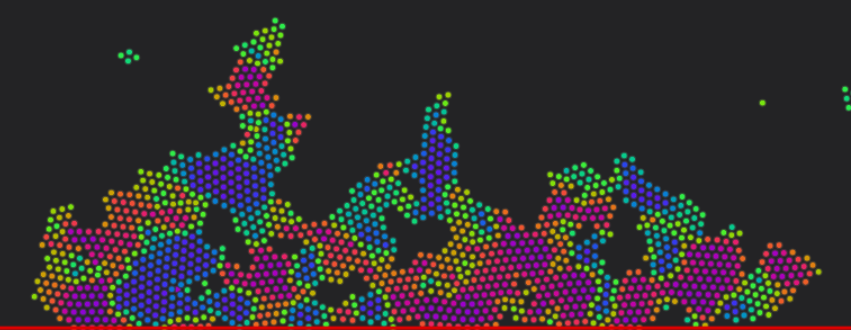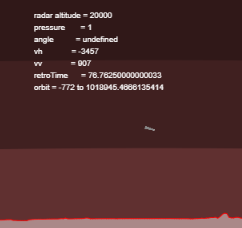﻿ Maths Exam

### Maths Exam

1. Expand the Brackets

 1 Expand the brackets to simplify the following:$$-3\left(-3P + -5y\right)$$Correct Answer $$9P + 15y$$ 2 Expand the brackets to simplify the following:$$5\left(-2a + 4A\right)$$Correct Answer $$-10a + 20A$$

2. Expand the Brackets

 1 Expand the brackets to simplify the following:$${\left(-4x + 2b\right)}^{2}$$Correct Answer $$16{x}^{2} - 16xb + 4{b}^{2}$$ 2 Expand the brackets to simplify the following:$${\left(4g + -4j\right)}^{2}$$Correct Answer $$16{g}^{2} - 32gj + 16{j}^{2}$$

3. Collect Like Terms

 1 Collect like terms to simplify the following:$$5a + 3Q + 1u + 1Q$$Correct Answer $$5a + 4Q + u$$ 2 Collect like terms to simplify the following:$$1X + 3S + (-l) + -5S$$Correct Answer $$X + -2S - l$$

 1 Simplify the following:$$\frac{-4x + 3}{-3x + -6} + \frac{4x + 6}{(-x) + -4}$$Correct Answer $$\frac{-8{x}^{2} + -29x - 48}{3{x}^{2} + 18x + 24}$$ 2 Simplify the following:$$\frac{2x + 4}{5x + 1} + \frac{-3x + 3}{-5x + -3}$$Correct Answer $$\frac{-25{x}^{2} + -14x - 9}{-25{x}^{2} - 20x - 3}$$

5. Evaluate the following equations.

 1 Find V to 3 significant figures in the following equation: $$V = \frac{-3 - x}{y - 6} - \sin\left(-3z\right),$$ Given that x = -4.0, y = -6.0, z = -4.0Correct Answer 0.453 2 Find B to 3 significant figures in the following equation: $$B = \frac{4z\sin\left(\cos\left(x\right)\right)}{\cos\left(y\right)},$$ Given that x = 1.0, y = 1.0, z = -3.0Correct Answer -11.4

6. Rearrange the following equation.

 1 Rearrange the following equation to get an expression for m: $$\frac{\left(5 - y\right)\exp\left(x + 6\right)}{\sqrt{z}} = \frac{z - 2}{\exp\left(x\right)} - \left(m + z\right)\sqrt{y},$$ Correct Answer $$m = \frac{\frac{z - 2}{\exp\left(x\right)} - \frac{\left(5 - y\right)\exp\left(x + 6\right)}{\sqrt{z}}}{\sqrt{y}} - z$$ 2 Rearrange the following equation to get an expression for m: $$\frac{\left(z - y\right)\cos\left(\frac{3}{y}\right)}{xz} = 2zm - \left(-4 - x\right)\left(x - 3\right),$$ Correct Answer $$m = \frac{\frac{\frac{\left(z - y\right)\cos\left(\frac{3}{y}\right)}{xz} + \left(-4 - x\right)\left(x - 3\right)}{2}}{z}$$

 Solve the following equation to get a value for x:$$6{x}^{2} + -2x = 5$$Correct Answer $$\frac{2 + \sqrt{124}}{12}$$ and $$\frac{2 - \sqrt{124}}{12}$$

8. Expand the Brackets

 1 Expand the brackets to simplify the following:$$-3\left((-c) + -2Y\right)$$Correct Answer $$3c + 6Y$$ 2 Expand the brackets to simplify the following:$$4\left(-3P + -6Y\right)$$Correct Answer $$-12P - 24Y$$

9. Expand the Brackets

 1 Expand the brackets to simplify the following:$${\left(5c + 2A\right)}^{2}$$Correct Answer $$25{c}^{2} + 20cA + 4{A}^{2}$$ 2 Expand the brackets to simplify the following:$${\left(-5e + 1Y\right)}^{2}$$Correct Answer $$25{e}^{2} - 10eY + {Y}^{2}$$

10. Collect Like Terms

 1 Collect like terms to simplify the following:$$3a + -6Q + 2l + -3Q$$Correct Answer $$3a + -9Q + 2l$$ 2 Collect like terms to simplify the following:$$-4R + 2y + 5Z + -3y$$Correct Answer $$-4R + (-y) + 5Z$$

## Three Crystal Structures

A ThreeJS model of three crystal structures, by Dr. Jennifer Martay.## Atomistic Simulation of Metals

This presents an interactive simulation of atoms making up a nanoscopic particle of metal.## Mars Lander

An interactive simulation of a Mars Lander trying to land on Mars. The term "interactive" here means that you have to write the software, in Javascript, that will land the probe.